# Convolutional Neural Networks Part 2¶

In :
from ipypublish import nb_setup


## Introduction¶

The previous chapter went over the basics of ConvNet design and implementation. In the current chapter we cover some advanced topics, including:

• Brief descriptions of some important ConvNets, with particular emphasis on those whose pre-trained models are made available in Keras
• Visualization of filters and Activation Maps in ConvNets and techniques to run a ConvNet backwards to generate images. These are used probe the responses of individual neurons and thus gain some understanding of how these systems work and arrive at their decisions.
• Using ConvNets in other Image Processing tasks such as Object Localization and Detection, Semantic Categorization etc.

The performance of ConvNets has improved in recent years due to several architectural changes, which include the following:

• Residual Connections
• Use of Small Filters
• Bottlenecking Layers
• Grouped Convolutions (also called Split-Transform-Merge)
• Depthwise Separable Convolutions
• Dispensing with the Pooling Layer
• Dispensing with Fully Connected Layers

Residual Connections are arguably the most important architectural enhancement made to ConvNets since their inception, and are covered in some detail in the following section. Even though proposed in the context of ConvNets, they are critical part of other Neural Network architectures, in particular Transformers.

### Residual Connections¶

In :
#convnet34
nb_setup.images_hconcat(["DL_images/convnet34.png"], width=700)

Out: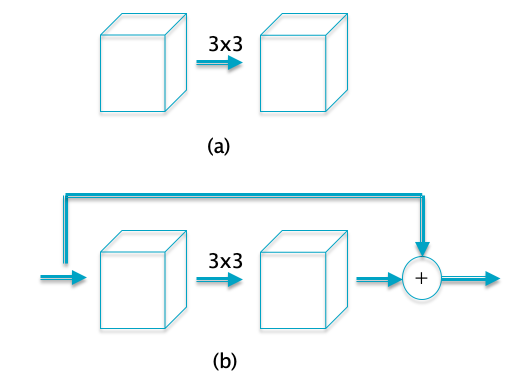Residual connections are illustrated in Part (b) of Figure convnet34. They were introduced as part of the ResNet model in 2015 and were shown to lead to large improvement in model performance by making possible trainable models with hundreds of layers. Prior to the discovery of Residual Connections, models with more 20-30 layers ran into the Vanishing Gradient problem during training, due to the gradient dying out as it was subject to multiple matrix multiplication during Backprop.

Part (a) of the figure shows a set of regular conolutional layers, Residual Connections feature a bypass connection from the input to the output such that the input signal is added to the output signal as shown in Part(b). This design clearly helps with gradient propagation, since the addition operation has the effect of taking the gradient at the output of the sub-network and propagating it without change to the input. The idea of Residual Connections is now widely used in DLN architectures, state of the art networks such DenseNet and Transformers include it as part of their design. We provide a deeper discussion of the benefits of Residual Connections in the section on ResNets later in this chapter.

### Small Filters in ConvNets¶

In :
#convnet11
nb_setup.images_hconcat(["DL_images/convnet11.png"], width=600)

Out: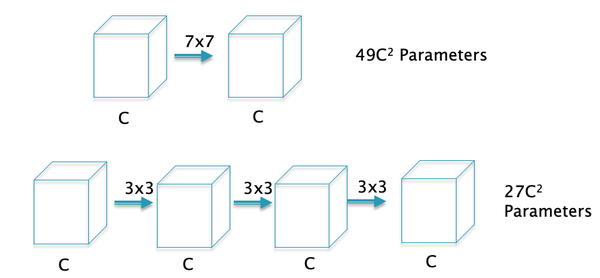s As ConvNet architectures have matured, one of the features that has changed over time is the filter design. Early ConvNets such as AlexNet used large $7 \times 7$ Filters in the first convolutional layer. However, a stack of $3 \times 3$ Filters is a superior design to the single $7 \times 7$ Filter for the following reasons (see Figure convnet11)):

• The stacked $3 \times 3$ design uses a smaller number of parameters: Using the formula for number of parameters in a ConvNet developed in Section SizingConvnets, it follows that the $7 \times 7$ Filter uses $C \times (7 \times 7 \times C + 1)=49 C^2 + C$ parameters (since each Filter uses $7 \times 7 \times C + 1$ parameters, and there are C such Filters). In comparison the stacked $3 \times 3$ design uses a total of $3 \times C \times (3 \times 3 \times C + 1)=27 C^2 + 3C$ parameters, i.e., a 45% decrease.

• The stacked $3 \times 3$ design incorporates more non-linearity, it has 4 ReLU Layers vs the 2 ReLU Layers in the $7 \times 7$ case, which increases the system's modeling capacity.

• The stacked $3 \times 3$ design results in less computation.

The better performance with smaller filters can be explained as follows: The job of a filter is to capture patterns within the Local Receptive Field and patterns at a finer level of granularity can be captured with smaller filters. Also as we stack up the layers, filters at the deeper levels progressively capture patterns within larger areas of the image. For example consider the case of the stacked $3 \times 3$ Filters in Figure convnet11: While the first $3 \times 3$ Filter scans patches of size $3 \times 3$ in the input image, it can be easily seen that the second $3 \times 3$ filter effectively scans patches of size $5 \times 5$, and the third $3 \times 3$ Filter scans patches of size $7 \times 7$ in the input image. Hence even though the filter size is unchanged as we move deeper into the network, they are able to capture patterns in progressively larger sections of the input image. This is illustrated in Figure convnet33 for the case of two layers of $3\times 3$ filters.

In :
#convnet33
nb_setup.images_hconcat(["DL_images/convnet33.png"], width=600)

Out: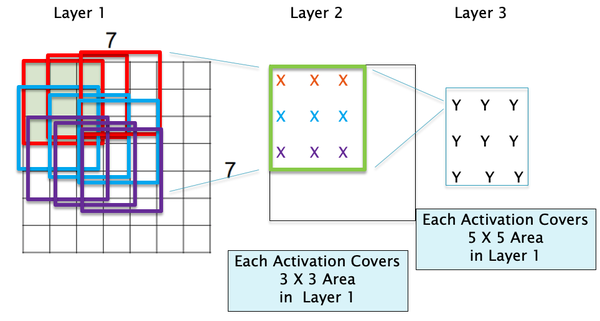### Bottlenecking using 1x1 Filters¶

In :
#cnn21
nb_setup.images_hconcat(["DL_images/cnn21.png"], width=600)

Out: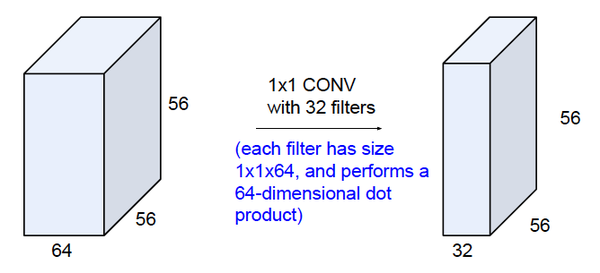Several modern ConvNets use Filters of size $1\times 1$. At first glance these may not make sense, but note that because of the depth dimension, a $1\times 1$ Filter still involves a dot product with weights associated with the depth dimension and the corresponding activations across them. As shown in Figure cnn21, this can be used to compress the number of layers in a ConvNet. This operation called 'bottlenecking', is very useful and is frequently used in modern ConvNets to reduce the number of parameters or the number of operations required.

In :
#convnet12
nb_setup.images_hconcat(["DL_images/convnet12.png"], width=600)

Out: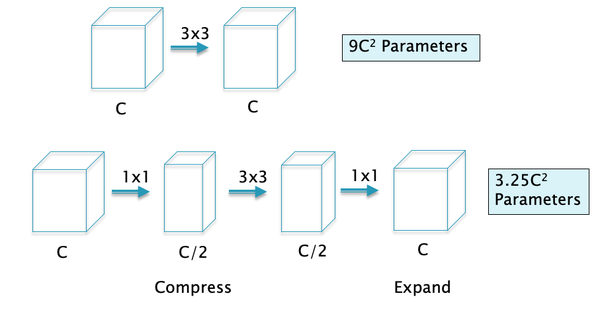Bottlenecking with $1 \times 1$ and $1 \times n$ filters, as illustrated in Figure convnet12: As shown, the first $1 \times 1$ filter is used in conjuction with a reduction in depth to $C/2$. The $3 \times 3$ filter then acts on this reduced depth layer, which gives us the reduction in parameters, and this is followed by another $1 \times 1$ filter that expands the depth back to $C$. It can the shown that this filter architecture reduces the number of parameters from $9 C^2$ to $3.25 C^2$ (ignoring the bias parameters). It can be easily shown that the number of computations also decreases from $9C^2 HW$ to $3.25C^2 HW$, where $H,W$ are the spatial dimensions of the ConvNet.

### Grouped Convolutions¶

In :
#convnet27
nb_setup.images_hconcat(["DL_images/convnet27.png"], width=800)

Out: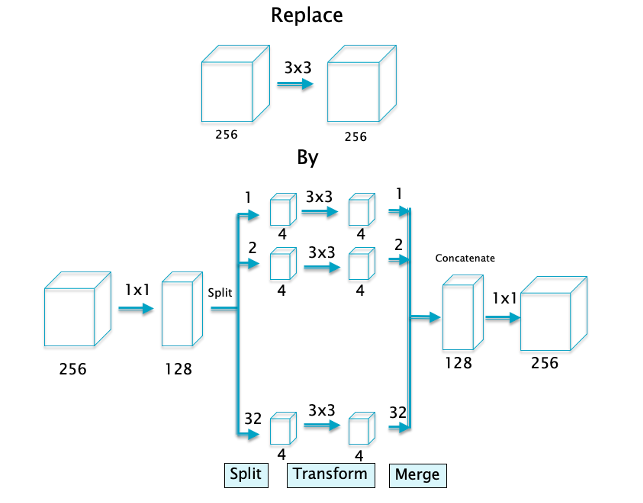Grouped Convolutions are illustrated in Figure convnet27. As shown, the regular $3\times 3$ convolution is replaced by the following:

• Step 1: Compress the input layers using a $1\times 1$ convolution from 256 layers to 128.
• Step 2: Split the output of Step 1 into 32 separate convolution layers, such that each layer 4 Activation Maps.
• Step 3: Use regular $3\times 3$ convolution on each of the 32 layers
• Step 4: Marge or Concatenate all 32 convolution layers to form a structure with 128 Activation Maps
• Step 5: Expand the output of step 4 to 256 Activation Maps with a $1\times 1$ convolution

Grouped Convolutions have been shown to improve the performance of ConvNets. It is not completely clear how they are able to do so, but it has been observed that the 4 Activation Maps in the smaller Convolutional Layers learn features that have a high degree of correlation. For example AlexNet, which was one of the earliest ConvNets, featured 2 groups due to memory constraints, and it was shown that Group 1 learnt black and white shapes while Group 2 learnt colored shapes. This functions as akind of regularizer and helps the network learn better features.

It can also be shown that the number of computations for the Grouped case decreases in inverse proportion to the number of groups and the size of the filter. This calculation is carried out detail in the next section.

### Depthwise Separable Convolutions¶

In :
#convnet28
nb_setup.images_hconcat(["DL_images/convnet28.png"], width=900)

Out: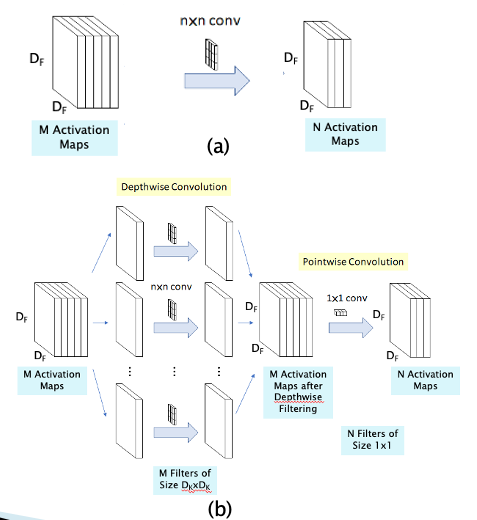A standard convolution both filters and combines inputs into a new set of outputs in one step. The depthwise separable convolution splits this into two layers, a separate layer for filtering and a separate layer for combining. This factorization has the effect of drastically reducing computation and model size. Hence they take the idea behind Grouped Convolutions to the limit by splitting up the M Activation Maps in the input layer into M separate single layer Activation Maps as shown in Figure convnet28. Each of these Activation Maps is then processed using a separate convolutional filter, and then combined together at the output. A simple $1\times 1$ convolution, is then used to create a linear combination of the output of the depthwise layer . Hence this design serializes the spatial and depthwise filtering operations of a regular convolution. An immediate benefit of doing this is a reduction in the number of computations (and the number of parameters), as shown next:

• The number of computations in a regular convolution shown in Part (a) is given by $D_K D_K\times MN\times D_F D_F$ where $D_K$ is the size of the filter, M is the depth of the input layer, N is the depth of the output layer and $D_F$ is the size of the Activation Map.
• The number of computations in the Depthwise Separable Convolution is given by $D_K D_K\times M\times D_F D_F + MN\times D_F D_F$

It follows that the ratio $R$ between the number of computations in the Depthwise Separable network and the Regular COnvNet is given by

$$R = {1\over N} + {1\over D_K^2}$$

For a typical filter size of $D_K= 3$, it follows that the number of computations in the Depthwise Separable network reduces by a factor of 9, which can be a significant reduction for training times that sometimes run into days. The idea of separating out the filtering into two parts, spatial and depthwise, has subsequently proven to be very influential, and underlies the type of filtering in a new model called Transformers, which we will study in a later chapter.

Depthwise Separable Convolutions are supported in Keras usong the SeparableConv2D command that implements all the operations shown in Part (b) of the figure.

## ConvNet Architectures¶

In :
#convnet15
nb_setup.images_hconcat(["DL_images/convnet15.png"], width=600)

Out: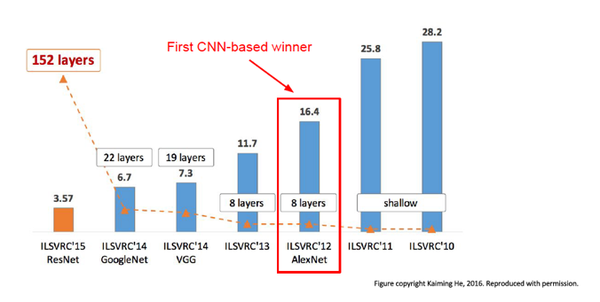We briefly survey ConvNet architectures starting with the first ConvNet, LeNet5, whose details were published in 1998. Starting with AlexNet in 2012, ConvNets have won the ImageNet Large Scale Visual Recognition Challenge (ILSVRC) image classification competition run by the Artificial Vision group at Stanford University. ILSVRC is based on a subset of the ImageNet database that has more than 1+ million hand labeled images, classified into 1,000 object categories with roughly 1,000 images in each category. In all there are 1.2 million training images, 50K validation images and 150K testing images. If an image has multiple objects, then the system is allowed to output upto 5 of the most probably object categories, and as long as the the "ground-truth" is one of the 5 regardless of rank, the classification is deemed successful (this is referred to as the top-5 error rate).

Figure convnet15 plots the top-5 error rate and (post-2010) the number of hidden layers in DLN architectures for the past seven years. Prior to 2012, the winning designs were based on traditional ML techniques using SVMs and Feature Extractors. In 2012 AlexNet won the competition, while bringing down the error rate signficantly, and inaugurated the modern era of Deep Learning. Since then, error rates have come down rapidly with each passing year, and currently stand at 3.57%, which is better than human-level classification performance. At the same time, the number of Hidden Layers has increased from the 8 layers used in AlexNet, to 152 layers in ResNet which was the winner in 2015. This is when the machines outpaced humans in accuracy and the competetion was stopped.

In this section our objective is to briefly describe the architecture of the winners from the last few years, namely: AlexNet (2012), ZFNet (2013), VGGNet (2014), Google InceptionNet (2014) and ResNet (2015). Note that VGGNet, InceptionNet and VGGNet are available as pre-trained models (on ImageNet) in Keras, for use in Transfer Learning. In addition we will go over a few other architectures whose pre-trained models are also included in Keras, namely XceptionNet, MobileNet, DenseNet and NASNet. The first three are "classical" ConvNet designs with multiple Convolutional, Pooling, and Dense layers arranged in a linear stack. The Google Inception Network and ResNet have diverged from some of the basic tenets of the first ConvNets, by adopting the Split/Transform/Merge and the Residual Connection designs respectively. In more recent years the focus has shifted towards coming up with ConvNets that have a much lower number of parameters, without sacrificing performance. The InceptionNet actually began this trend, and MobileNet also belongs to this category of models.

These following architectures are described in greater detail next:

• LeNet (1998)
• AlexNet (2012)
• ZFNet (2013)
• VGGNet (2014)
• InceptionNet (2014)
• ResNet (2015)
• Xception (2016)
• DenseNet (2017)
• MobileNet (2017)
• NasNet (2018)

The table below which is taken from Keras Documentation summarizes the size, performance, number of parameters and depth of these models. The performance is with respect to the ImageNet dataset, with the Top-1 Accuracy being the performance for the best predition and the Top-5 Accuracy being the performance for the case when the Ground Truth is among the top 5 predictions.

In :
#ConvNetPerformance
nb_setup.images_hconcat(["DL_images/convnet35.png"], width=600)

Out: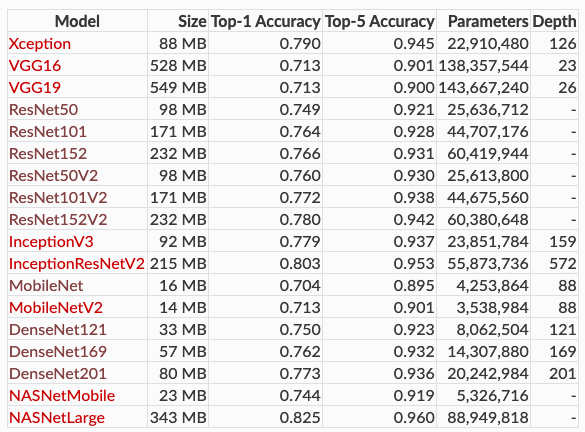### LeNet5 (1998)¶

LeNet5 was the first ConvNet, it was designed by Yann LeCun and his team at Bell Labs, see Lecun, Bottou, Bengio, Haffner (1998). It had all the elements that one finds in a modern ConvNet, including Convolutional and Pooling Layers, along with the Backprop algorithm for training (Figure LeNet5)). It only had two Convolutional layers, which was a reflection of the smaller training datsets and processing capabilities available at that time. LeCun et.al. used the system for handwritten signature detection in checks, and it was successfully deployed commercially for this purpose.

In :
#LeNet5
nb_setup.images_hconcat(["DL_images/LeNet5.png"], width=900)

Out: